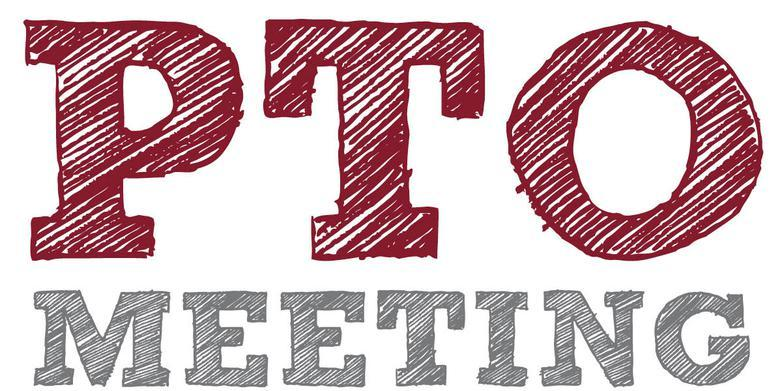/* styles */ Hi DC Community!

# The Desert Cove eNews is sent on a MONTHLY basis.

If you do not wish to receive the Desert Cove PTO eNews,
please click 'Unsubscribe' at the bottom of this emailDECEMBER 2018

▪ Dec 3-9: Computer Science Week! #HourOfCode
▪ Dec 4: EARLY RELEASE
▪ Dec 6: DC Spirit Night at MyPie 3PM - 9PM
▪ Dec 11: PTO Meeting @ 6PM
▪ Dec 14: 6th Grade Yearbook Dedications Due
▪ Dec 19: Staff Holiday Luncheon
▪ Dec 24 - Jan 4: NO SCHOOL
 ▪ Dec 3-9: Computer Science Week! #HourOfCode
 ▪ Dec 4: EARLY RELEASE
 ▪ Dec 6: DC Spirit Night at MyPie 3PM - 9PM
 ▪ Dec 11: PTO Meeting @ 6PM
 ▪ Dec 14: 6th Grade Yearbook Dedications Due
 ▪ Dec 19: Staff Holiday Luncheon
 ▪ Dec 24 - Jan 4: NO SCHOOL

 table div table+table+table+table+table+table div table{width:100%;padding:0}table div table+table+table+table+table+table div table img{width:96.23%;padding:0;float:none}table div table+table+table+table+table+table div table td{width:100%;padding:0 1.88% 18px}/* styles */table.module-6{width:99.06%;padding:0}table div table+table+table+table+table+table+table div table{width:99.06%;float:none;margin-left:auto;margin-right:auto;padding:0}table div table+table+table+table+table+table+table div table a{border:0 none;text-decoration:none}table div table+table+table+table+table+table+table div table img{width:100%!important;border:0 none;text-decoration:none}table div table+table+table+table+table+table+table div table td{width:100%;padding:0}/* styles */
 table div table+table+table+table+table+table+table+table div table td,table.module-7{width:100%;padding:0}table div table+table+table+table+table+table+table+table div table{width:100%;float:none;margin-left:auto;margin-right:auto;padding:0}table div table+table+table+table+table+table+table+table div table a{border:0 none;text-decoration:none}table div table+table+table+table+table+table+table+table div table img{width:100%!important;border:0 none;text-decoration:none}/* styles */
 table div table+table+table+table+table+table+table+table+table div table{width:100%;padding:0}table div table+table+table+table+table+table+table+table+table div table img{width:96.23%;padding:0;float:none}table div table+table+table+table+table+table+table+table+table div table td{width:100%;padding:0 1.88% 18px}/* styles */# Join the LARGEST learning event in history and set aside time for an #HourOfCode this week!

 table div table+table+table+table+table+table+table+table+table+table+table+table+table div table td,table.module-12{width:100%;padding:0}table div table+table+table+table+table+table+table+table+table+table+table+table+table div table{width:100%;float:none;margin-left:auto;margin-right:auto;padding:0}table div table+table+table+table+table+table+table+table+table+table+table+table+table div table a{border:0 none;text-decoration:none}table div table+table+table+table+table+table+table+table+table+table+table+table+table div table img{width:100%!important;border:0 none;text-decoration:none}/* styles */
 table div table+table+table+table+table+table+table+table+table+table+table+table+table+table div table{width:100%;padding:0}table div table+table+table+table+table+table+table+table+table+table+table+table+table+table div table img{width:96.23%;padding:0;float:none}table div table+table+table+table+table+table+table+table+table+table+table+table+table+table div table td{width:100%;padding:0 1.88% 18px}/* styles */## DEC 6: DC SPIRIT NIGHT @ MY PIE3:00pm-8:00pm at 12601 N. Tatum Blvd

 table div table+table+table+table+table+table+table+table+table+table+table+table+table+table+table+table div table td,table.module-15{width:100%;padding:0}table div table+table+table+table+table+table+table+table+table+table+table+table+table+table+table+table div table{width:100%;float:none;margin-left:auto;margin-right:auto;padding:0}table div table+table+table+table+table+table+table+table+table+table+table+table+table+table+table+table div table a{border:0 none;text-decoration:none}table div table+table+table+table+table+table+table+table+table+table+table+table+table+table+table+table div table img{width:100%!important;border:0 none;text-decoration:none}/* styles */
 table div table+table+table+table+table+table+table+table+table+table+table+table+table+table+table+table+table div table{width:100%;padding:0}table div table+table+table+table+table+table+table+table+table+table+table+table+table+table+table+table+table div table img{width:96.23%;padding:0;float:none}table div table+table+table+table+table+table+table+table+table+table+table+table+table+table+table+table+table div table td{width:100%;padding:0 1.88% 18px}/* styles */## DEC 11: PTO MEETING# FREE Pizza & Childcare provided!

Can't make the meeting? No worries, Meeting Minutes are posted on our website:

www.desertcovepto.org/meetings

 table div table+table+table+table+table+table+table+table+table+table+table+table+table+table+table+table+table+table+table+table div table{width:100%;padding:0}table div table+table+table+table+table+table+table+table+table+table+table+table+table+table+table+table+table+table+table+table div table img{width:96.23%;padding:0;float:none}table div table+table+table+table+table+table+table+table+table+table+table+table+table+table+table+table+table+table+table+table div table td{width:100%;padding:0 1.88% 18px}/* styles */## DEC 14: 6TH GRADE YEARBOOK DEDICATIONS DUE

 /* styles */ The DCES Yearbook Staff is accepting dedications and/or a photograph of your 6th grader for the back of the yearbook this year. It is FREE to participate. Send the dedications via email to: dcesyearbookdedications@gmail.com PLEASE INCLUDE THE FOLLOWING IN THE EMAIL: * Child's First AND Last Name * 6th Grade Teacher's Name * Short Dedication (50 word maximum) -- Not sure what to write? Here are some samples! * ONE High Quality Photograph (OPTIONAL) IMPORTANT: Late submissions WILL NOT make it into the yearbook due to publishing timelines
 table div table+table+table+table+table+table+table+table+table+table+table+table+table+table+table+table+table+table+table+table+table+table+table div table{width:100%;padding:0}table div table+table+table+table+table+table+table+table+table+table+table+table+table+table+table+table+table+table+table+table+table+table+table div table img{width:96.23%;padding:0;float:none}table div table+table+table+table+table+table+table+table+table+table+table+table+table+table+table+table+table+table+table+table+table+table+table div table td{width:100%;padding:0 1.88% 18px}/* styles */We have created a page on the PTO website where you can access the following communications:

Desert Cove PTO eNews archives
Desert Cove Principal News
PV School District eNews
United Parent Council eNews